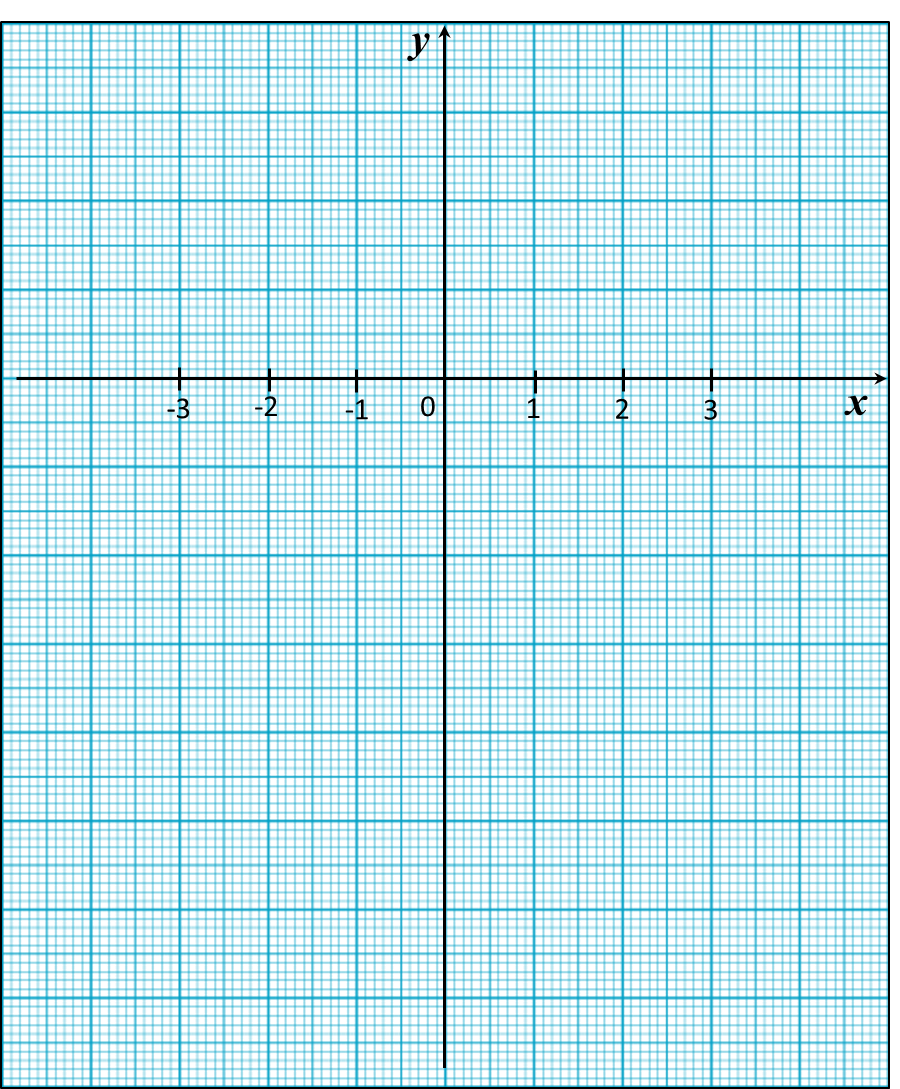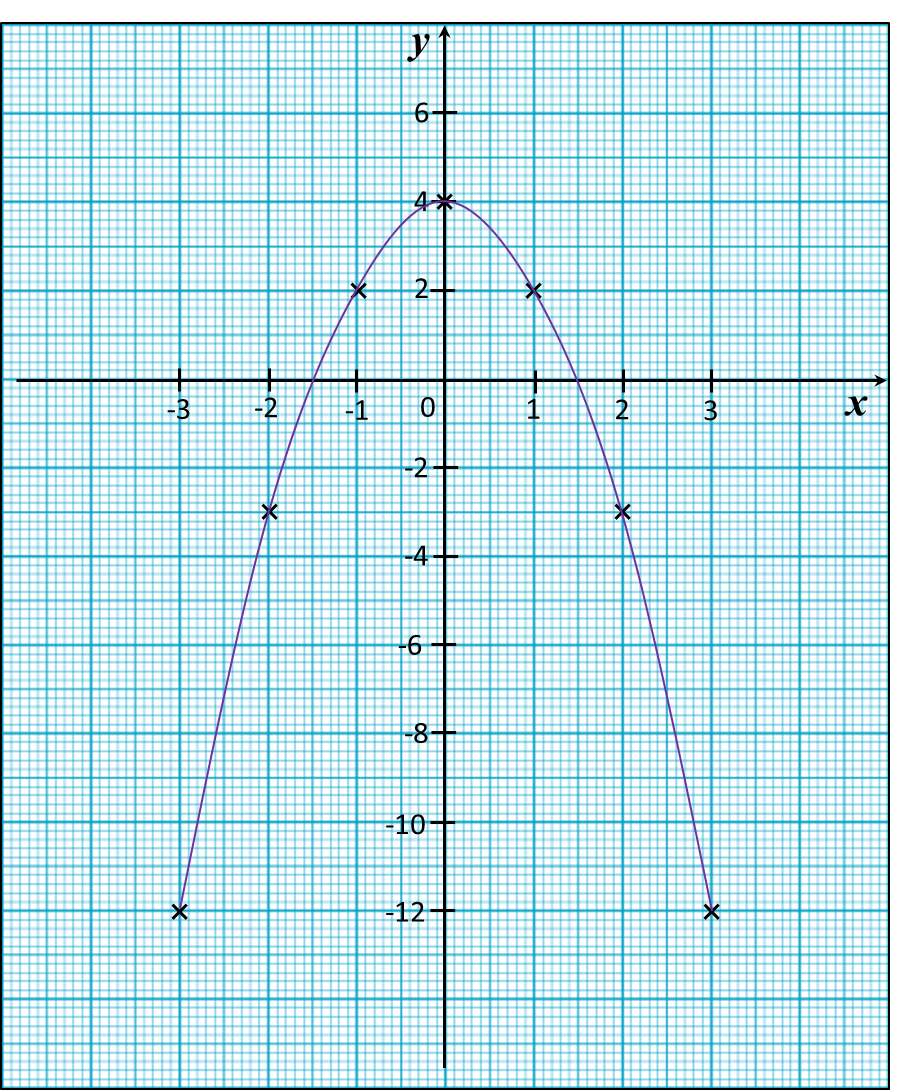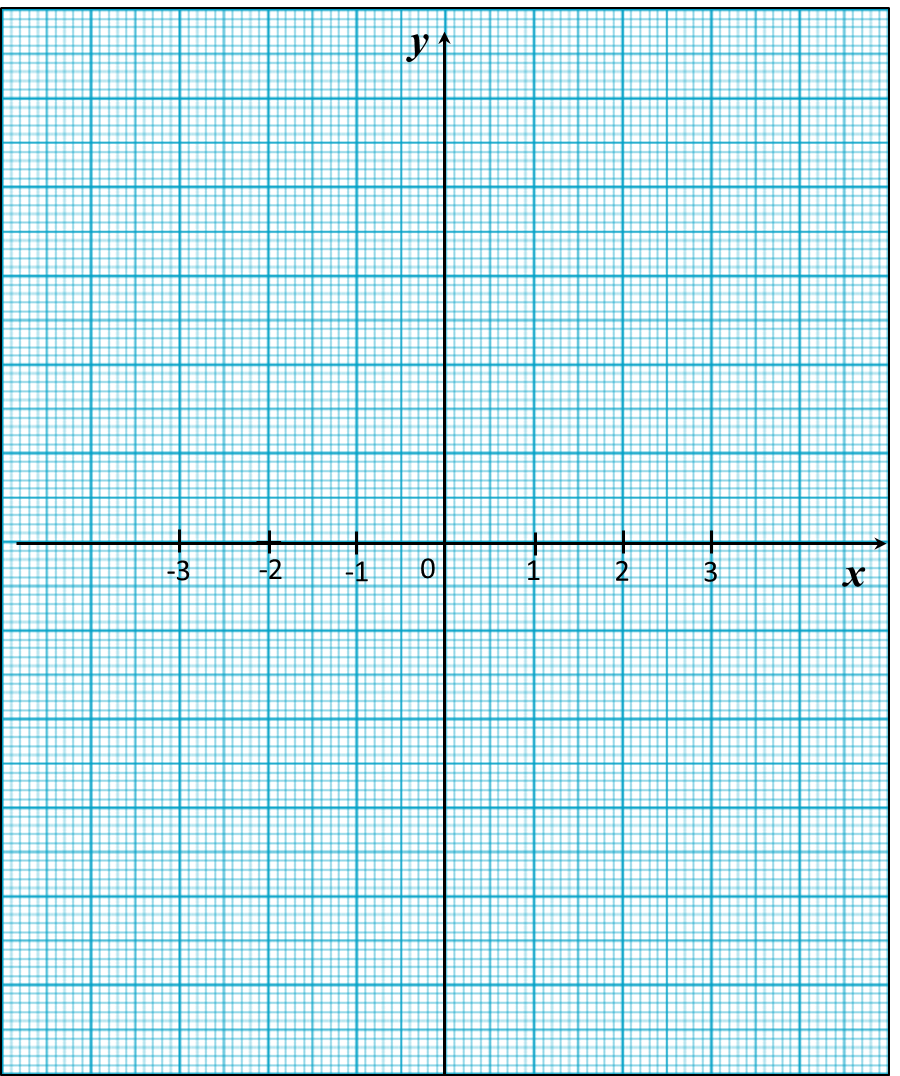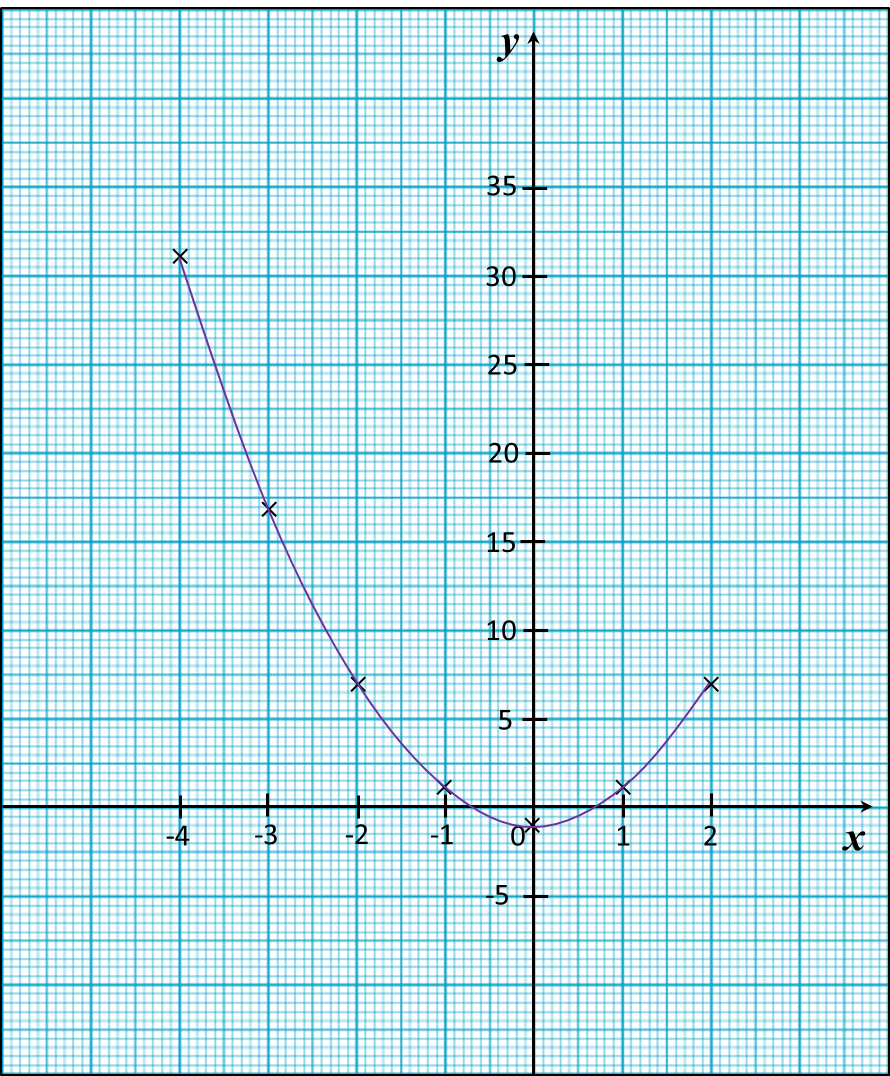# 13.2.3 Graphs of Functions, PT3 Focus Practice

Question 7:
Use graph paper to answer this question.
Table below shows the values of two variables, x and y, of a function.The x-axis and the y-axis are provided on the graph paper on the answer space.
(a) By using a scale of 2 cm to 5 units, complete and label the y-axis.
(b) Based on the table above, plot the points on the graph paper.
(c) Hence, draw the graph of the function.Solution:Question 8:
Use graph paper to answer this question.
Table below shows the values of two variables, x and y, of a function.The x-axis and the y-axis are provided on the graph paper on the answer space.
(a) By using a scale of 2 cm to 2 units, complete and label the y-axis.
(b) Based on the table above, plot the points on the graph paper.
(c) Hence, draw the graph of the function.Solution:# 13.2.2 Graphs of Functions, PT3 Focus Practice

 
 
13.2.2 Graphs of Functions, PT3 Focus Practice
 
 
Question 4:
 
Use graph paper to answer this question.
 
Table below shows the values of two variables, x and y, of a function.
 

 
 
   x     –3     –2     –1     0     1     2     3     y     –19     –3     1     –1     –3     1     17  
 
 
The x-axis and the y-axis are provided on the graph paper on the answer space.
 
(a)  By using a scale of 2 cm to 5 units, complete and label the y-axis.
 
(b) Based on the table above, plot the points on the graph paper.
 
(c)  Hence, draw the graph of the function.
 
 

 Answer:Solution:Question 5:
 
Use graph paper to answer this question.
 
Table below shows the values of two variables, x and y, of a function.
 

 
 
   x     –4     –3     –2     –1     0     1     2     y     31     17     7     1     –1     1     7  
 
 
The x-axis and the y-axis are provided on the graph paper on the answer space.
 
(a)  By using a scale of 2 cm to 5 units, complete and label the y-axis.
 
(b)   Based on the table above, plot the points on the graph paper.
 
(c)  Hence, draw the graph of the function.Solution:Question 6:
(a) Complete table below in the answer space for the equation L = x2 + 5x by writing the value of L when x = 2.
(b) Use graph paper to answer this part of the question. You may use a flexible curve rule.
By using a scale of 2 cm to 1 unit on the x-axis and 2 cm to 5 units on the y-axis, draw the graph of L = x2 + 5x for 0 ≤ x ≤ 4.Solution:
(a)
When x = 2
L = 22 + 5(2)
= 4 + 10
= 14

(b)# 13.2.1 Graphs of Functions, PT3 Focus Practice

13.2.1 Graphs of Functions, PT3 Focus Practice

Question 1:

In the figure shown, when x = 4, the value of y is

Solution:

is the point on the graph where x = 4.
Hence, when x = 4, y = 5.

Question 2:
Diagram shows the graph of a function on a Cartesian plane.

The equation that represents the function is

Solution:
Use points (0, 6) and (-3, 0) to determine the function.

 Function Value of y when x = 0 x = –3 y = x + 3 3 0 y = x – 3 –3 –6 y = 2x + 6 6 0 (correct) y = 2x – 6 –6 –12

Question 3:
Table below shows the values of variables x and y of a function.

 x –2 0 1 y –6 2 3
Which of the following functions is satisfied by the ordered pair?
A = 7x + 2
B = x3 + 2
C = x + x2 – 2
D = x2 + 2

Solution:
Only y = x3 + 2 is satisfied by all the ordered pairs.
(i) –6 = (–2)3 + 2
(ii)   2 = 03 + 2
(iii)  3 = 13 + 2

# 13.1 Graphs of Functions

13.1 Graphs of Functions

13.1.1 Functions
1.   Function is a relationship that expresses a variable (dependent) in terms of another variable (independent).

2.   is a function of x if and only if every value of x corresponds to only one value of y.

13.1.2  Graphs of Functions
1.   A graph of function is a representation of the function as one or more lines on a coordinate plane.
Example:

2.
When drawing graphs of functions, it is best to observe these few points:
· choose suitable scales for both axes.
· use at least three points to draw a straight line to ensure accuracy.
· use at least five points to draw a curve to ensure accuracy.